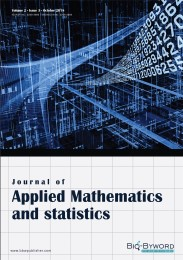Journal of Applied Mathematics and Statistics（JAMS） is a peer-reviewed &Open access journal on all areas of applied mathematics and statistics that intends to solve problems in engineering, sciences, and business through mathematical, computational and statistical methods. Topics of interest include, but are not limited to: applied mechanics, approximation theory, computational simulation, control, differential equations, dynamics, inverse problems, modeling, numerical analysis, optimization, probabilistic and statistical methods, stochastic processes. Research papers may be in any area of applied mathematics and statistics, with special emphasis on new mathematical ideas, relevant to modelling and analysis in modern science and technology, and the development of interesting mathematical methods of wide applicability.The topics related to this journal include but are not limited to:Scientific computing、Computer science、Operations research、Statistics、Actuarial science、Control theory、Computational logic、Coding theory、Dynamical systems、Discrete optimization、Discrete applied mathematics、Computational mathematics、Differential equations、Experimental mathematics、Fuzzy theory with applications、Linear and nonlinear programming、Mathematical biology、Numerical analysis、Numerical computation、Theory of computation、Theory of algorithms.